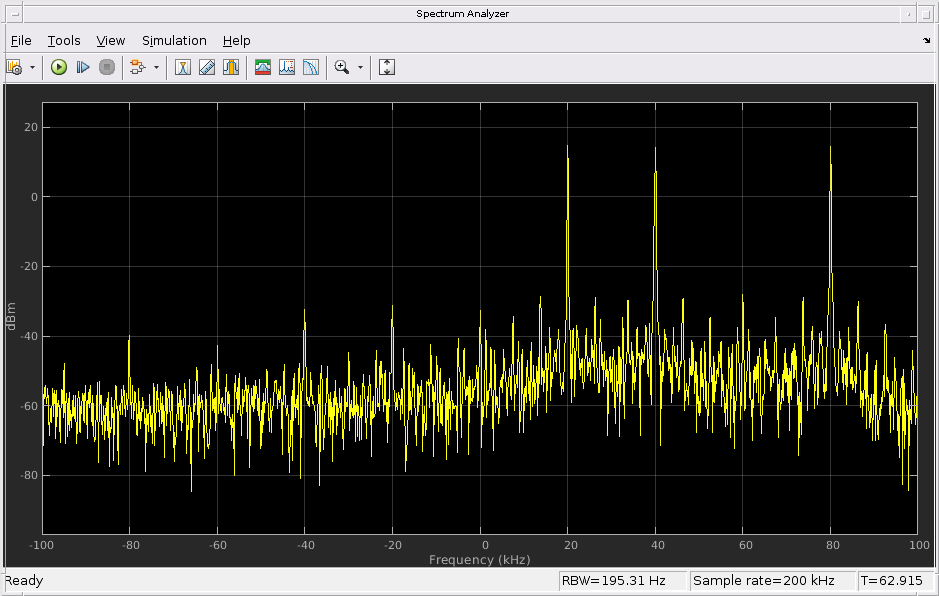This example shows how to synchronize both the baseband sample rate and the center frequency of two PlutoSDRs by using the Frequency correction parameter of the ADALM-PLUTO Radio Receiver block.

The baseband sample rate and the center frequency of a PlutoSDR are physically derived from the same oscillator. Although each PlutoSDR is factory-calibrated to use appropriate PLL settings to digitally compensate for component variations of the oscillator, the compensation is imperfect because of quantization effects and changes in operating conditions (temperature in particular). Consequently, two PlutoSDRs will operate at slightly different baseband sample rates and center frequencies even if the corresponding System objects or Simulink blocks for the two PlutoSDRs use the same baseband sample rate and the same center frequency. To solve this problem, the Frequency correction parameter of the ADALM-PLUTO Radio Receiver block can be used.

The Frequency correction parameter specifies the parts-per-million change to the baseband sample rate and the center frequency. The default value is 0, which means that the radio will use its factory-calibrated PLL settings. In this example, we treat one PlutoSDR, the transmitter, as a source with accurate baseband sample rate and center frequency. We use the signal received by another PlutoSDR, the receiver, to estimate the right value of Frequency correction for the receiver block, and show that when Frequency correction is set appropriately, the frequency of the received tone will be very close to 80 kHz, the frequency of the transmitted tone.

Refer to the Getting Started documentation for details on configuring your host computer to work with the PlutoSDR.

Algorithm for Estimating Frequency Correction

Consider a signal transmitted from one PlutoSDR to another PlutoSDR. Letand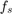be the center frequency and the baseband sample rate of the transmitter. Letand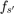be the center frequency and the baseband sample rate of the receiver. Assume that the oscillator of the transmitter has not drifted and the oscillator of the receiver has drifted by a factor, i.e.and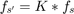whereis a number very close to 1.

A tone at baseband frequencyin the transmitted signal will appear as a tone at baseband frequencyin the receiver, where. The termin the numerator is due to the mismatch in center frequencies. The scaling factorin the denominator is due to the mismatch in baseband sample rates. Hence, we can solve forwith,, and..

To remove the frequency offset between the transmitter and the receiver, we need to scale the baseband sample rate and the center frequency of the receiver by a factor of. Let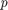be the parts-per-million change to the baseband sample rate and the center frequency of the receiver. Then. Therefore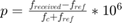.

Set up the Transmitter and the Receiver

We use one PlutoSDR to transmit three tones at 20, 40, and 80 kHz. The tone at 80 kHz is used to estimate the value for Frequency correction for the receiver. The tones at 20 and 40 kHz are only used to help visualize the spectrum.

% Set up parameters and signals
sampleRate = 200e3;
centerFreq = 2.42e9;
fRef = 80e3;
s1 = exp(1j*2*pi*20e3*[0:10000-1]'/sampleRate);  % 20 kHz
s2 = exp(1j*2*pi*40e3*[0:10000-1]'/sampleRate);  % 40 kHz
s3 = exp(1j*2*pi*fRef*[0:10000-1]'/sampleRate);  % 80 kHz
s = s1 + s2 + s3;
s = 0.6*s/max(abs(s)); % Scale signal to avoid clipping in the time domain

% Set up the transmitter
% Use the default value of 0 for FrequencyCorrection, which corresponds to
% the factory-calibrated condition
tx = sdrtx('Pluto', 'RadioID', 'usb:1', 'CenterFrequency', centerFreq, ...
'BasebandSampleRate', sampleRate, 'Gain', 0, ...
% Use the info method to show the actual values of various hardware-related
% properties
% Send signals
disp('Send 3 tones at 20, 40, and 80 kHz');
transmitRepeat(tx, s);

% Run the receiver. The detected frequency may be far from 80 kHz
disp(['The detected frequency is ' num2str(peakFreq/1000) ' kHz.']);
## Establishing connection to hardware. This process can take several seconds.

struct with fields:

Status: 'Full information'
CenterFrequency: 2.4200e+09
BasebandSampleRate: 200000
SerialNum: '1000002355237309002300260902167028'
Gain: 0
ExpectedFirmwareVersion: "0.30"
HardwareVersion: "A0"
FrequencyCorrection: 0

Send 3 tones at 20, 40, and 80 kHz
## Establishing connection to hardware. This process can take several seconds.
## Waveform transmission has started successfully and will repeat indefinitely.
## Call the release method to stop the transmission.
## Establishing connection to hardware. This process can take several seconds.
The detected frequency is 68.0386 kHz.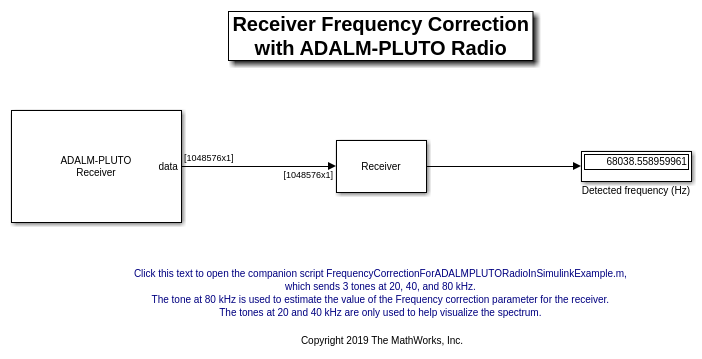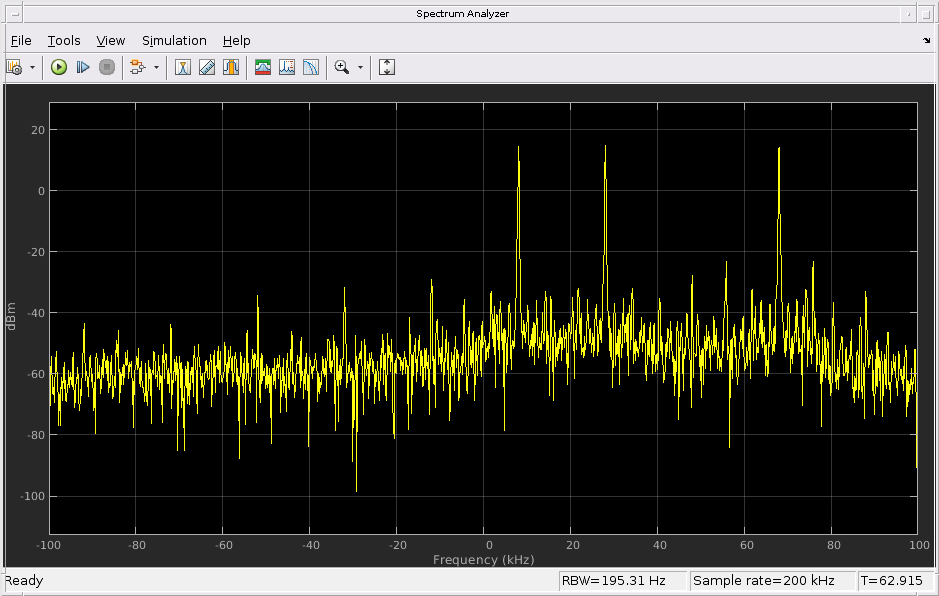Estimate the Frequency Correction Value

additionalCorrection = (peakFreq - fRef) / (centerFreq + fRef) * 1e6;

Apply the Frequency Correction Value

Since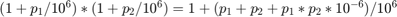, applying two changesandsuccessively is equivalent to applying a single change of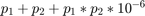with respect to the factory-calibrated condition.

currentFrequencyCorrection = str2double( ...
'FrequencyCorrection'))

newFrequencyCorrection = currentFrequencyCorrection + additionalCorrection + ...

'FrequencyCorrection',num2str(newFrequencyCorrection,'%.12f'))
currentFrequencyCorrection =

0

newFrequencyCorrection =

-4.9426

Verify the Results

Run the receiver again. The detected frequency should be closer to 80 kHz.

disp(['The detected frequency is ' num2str(peakFreq/1000) ' kHz.']);